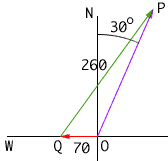Subject: Vector Problem Please can you help?! I understand vectors generally but I can't get the correct answer to this one. Maybe my diagram is wrong, I don't know. This is the problem: An aircraft can fly 260km/h in still air and the wind is blowing at 70km/h towards the West. In what direction should the aircraft head so that its actual velocity is on a bearing of 030 degrees? Many thanks for any help you can give me, Ben Hi Ben, I had to do some searching to find what "a bearing of 030 degrees" means but I think it is the angle from north, measures clockwise. If this is the correct meaning then the diagram I get isSince angle NOP is 30o, angle QOP is 120o. I would then use the law of sines to find the angle OPQ and hence you can determine the angle PQO which is the direction the aircraft should head. Cheers, Harley Go to Math Central